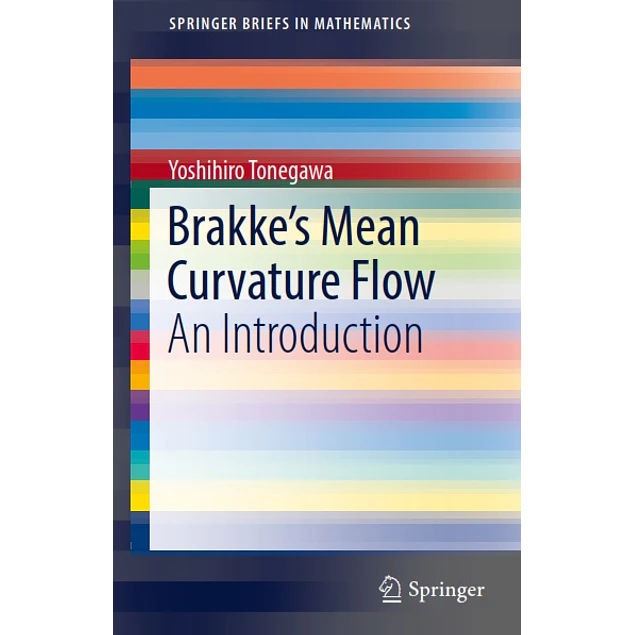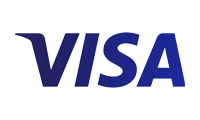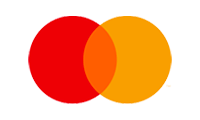## Brakke's Mean Curvature Flow: An Introduction

20.00\$# Brakke's Mean Curvature Flow: An Introduction

20.00\$

1st ed. 2019 Edition

by Yoshihiro Tonegawa (Author)

This book explains the notion of Brakke’s mean curvature flow and its existence and regularity theories without assuming familiarity with geometric measure theory. The focus of study is a time-parameterized family of k-dimensional surfaces in the n-dimensional Euclidean space (1 ≤ n). The family is the mean curvature flow if the velocity of motion of surfaces is given by the mean curvature at each point and time. It is one of the simplest and most important geometric evolution problems with a strong connection to minimal surface theory. In fact, equilibrium of mean curvature flow corresponds precisely to minimal surface. Brakke’s mean curvature flow was first introduced in 1978 as a mathematical model describing the motion of grain boundaries in an annealing pure metal. The grain boundaries move by the mean curvature flow while retaining singularities such as triple junction points. By using a notion of generalized surface called a varifold from geometric measure theory which allows the presence of singularities, Brakke successfully gave it a definition and presented its existence and regularity theories. Recently, the author provided a complete proof of Brakke’s existence and regularity theorems, which form the content of the latter half of the book. The regularity theorem is also a natural generalization of Allard’s regularity theorem, which is a fundamental regularity result for minimal surfaces and for surfaces with bounded mean curvature. By carefully presenting a minimal amount of mathematical tools, often only with intuitive explanation, this book serves as a good starting point for the study of this fascinating object as well as a comprehensive introduction to other important notions from geometric measure theory.

Year:
2019
Pages:
108
Language:
English
Format:
PDF
Size:
2 M
Publisher:
Springer
ISBN-10:
9811370745
ISBN-13:
978-9811370748
Tag:
Brakke's Mean Curvature Flow: An Introduction
##### Contact
••••